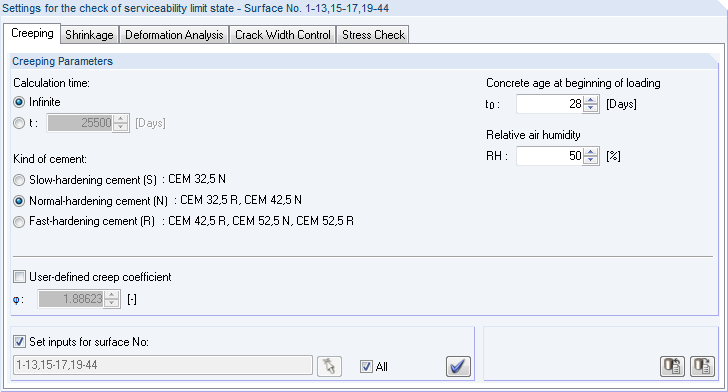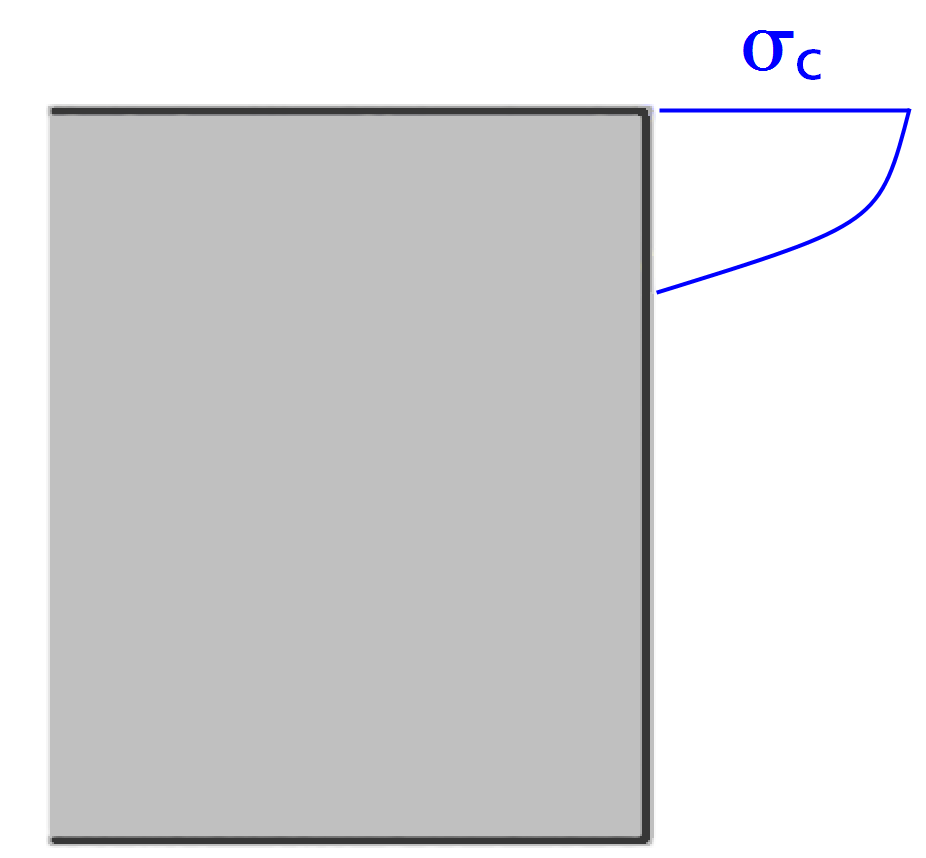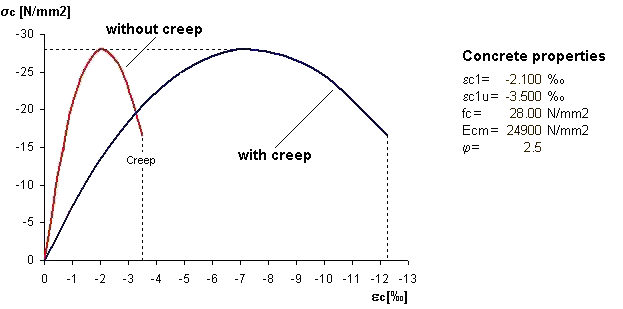# RF-CONCRETE Surfaces – Online Manual Version 5

Online manuals, introductory examples, tutorials, and other documentation.

# 2.8.4.1 Considering creep

#### Considering creep

Creep describes the time-dependent deformation of the concrete with loading within a particular period of time. The essential influence values are similar to those of shrinkage (see chapter 2.8.4.2). Additionally, the so-called "creep-producing stress" has a considerable effect on the creep deformations.

Attention must be paid to the load duration, the time of load application, as well as the extent of the loading. Creep is taken into account by the creep coefficient φ(t,t0) at the point of time t.

In RF-CONCRETE Surfaces, the specifications for determining the creep coefficient are set in window  1.3 Surfaces. In it, you can specify the concrete's age at the considered point of time and at the beginning of loading, the relative air humidity, as well as the type of cement. Based on these specifications, the program determines the creep coefficient φ.Figure 2.144 Window 1.3 Surfaces, Creeping tab

We now will briefly look at the determination of the creep coefficient φ according to EN 1992-1-1, clause 3.1.4. Using the following equations requires the creep-producing stress σc of the acting permanent load to not exceed the following value.

where

 fckj cylinder compressive strength of the concrete at the point of time when the creep-producing stress is appliedFigure 2.145 Creep-producing stress σc

Under the assumption of a linear creep behavior (σc < 0.45 ⋅ fckj), the concrete's creep can be determined through a reduction of the concrete's modulus of elasticity.

where

 Ecm mean modulus of elasticity according to EN 1992-1-1, Table 3.1 φ (t,t0) creep coefficient t age of concrete at relevant point of time in days t0 age of concrete when load application starts in days

According to EN 1992-1-1, clause 3.1.4, the creep coefficient φ(t,t0) at the analyzed point of time t can be calculated as follows.

where

 RH relative humidity [%] effective component thickness [mm] (for surfaces: h0 = h)Ac  cross-section areau    cross-section perimeter adjustment factorfcm  mean value of cylinder compressive strength adjustment factor coefficient for considering the concrete compressive strength coefficient for considering the age of concrete

 coefficient for considering the load duration age of concrete at relevant point of time in days age of concrete when load application starts in days

 relative humidity [%] effective component thickness [mm] adjustment factor

The influence of the type of cement on the concrete's creep coefficient can be taken into account by modifying the load application age t0 with the following equation:

where

 t0 = tT effective age of concrete when load application starts while taking the influence of temperature into account α exponent depending on type of cement: -1 : slow-hardening cements (S) (32,5)0 : normal- or rapid-hardening cements (N) (32,5 R; 42,5)1 : rapid-hardening, high-strength cements (R) (42,5 R; 52,5)
Considering creep in the calculation

If the strains at the point of time t = 0 as well as at a later point of time t are known, it is possible to determine the creep coefficient φ for a calculational consideration in the model.

This equation is rearranged to the strain at the point of time t. Thus, we get the following relation, which is valid for uniform stresses (cf. Equation 2.96):

For stresses higher than approximately 0.4 ⋅ fck, the strains increase disproportionately, resulting in the loss of the linearly assumed reference.

The calculation in RF-CONCRETE NL uses a common solution that is reasonable for construction purposes. The stress-strain diagram of the concrete is distorted by the factor (1 + φ).Figure 2.146 Distortion of the stress-strain relation for determining the creep effect

When taking creep into account, uniform creep-producing stresses are assumed during the period of load application, as can be seen in Figure 2.146. Because of neglected stress redistributions, the deformation is slightly overestimated due to this assumption. The stress reduction without a change in strain (relaxation) is only taken into account to a limited degree in this model. If we assume a linear elastic behavior, a proportionality could be presumed and the horizontal distortion would also reflect the relaxation at a ratio of (1 + φ). This correlation, however, is lost for the nonlinear stress-strain relationship.

Thus, it becomes clear that this procedure must be understood as an approximation. Therefore, a reduction of the stresses due to relaxation as well as nonlinear creep cannot or can only be approximately represented.